# Random Numbers in Excel

Excel has two very useful functions when it comes to generating random numbers. RAND and RANDBETWEEN.

### Rand

The RAND function generates a random decimal number between 0 and 1.

1. Select cell A1.

2. Type =RAND() and press Enter. The RAND function takes no arguments.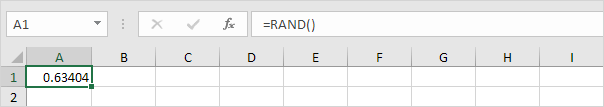3. To generate a list of random numbers, select cell A1, click on the lower right corner of cell A1 and drag it down.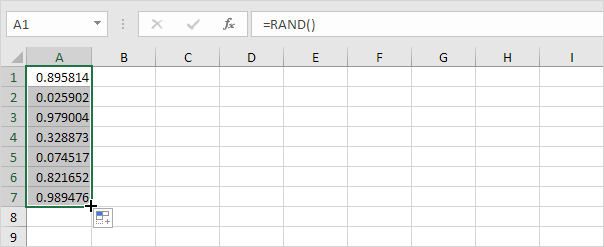Note that cell A1 has changed. That is because random numbers change every time a cell on the sheet is calculated.

4. If you don't want this, simply copy the random numbers and paste them as values.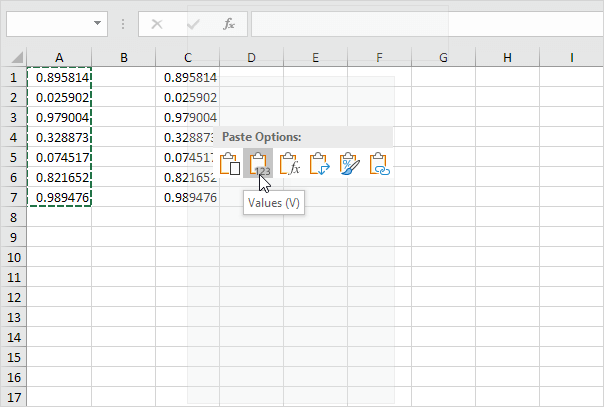5. Select cell C1 and look at the formula bar. This cell holds a value now and not the RAND function.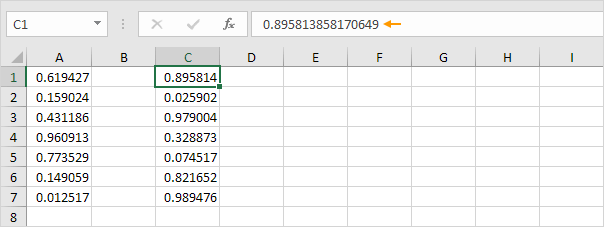### RandBetween

The RANDBETWEEN function generates a random whole number between two boundaries.

1. Select cell A1.

2. Type RANDBETWEEN(50,75) and press Enter.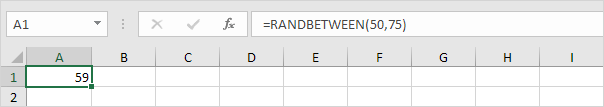3. If you want to generate random decimal numbers between 50 and 75, modify the RAND function as follows: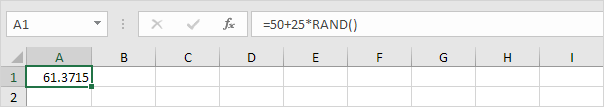### RandArray

If you have Excel 365 or Excel 2021, you can use the magic RANDARRAY function.

1. By default, the RANDARRAY function generates random decimal numbers between 0 and 1. The array below consists of 5 rows and 2 columns.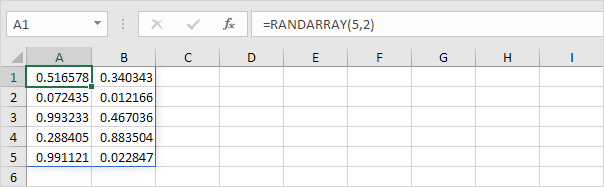Note: this dynamic array function, entered into cell A1, fills multiple cells. Wow! This behavior in Excel 365/2021 is called spilling.

2. The RANDARRAY function below generates an array of integers, 10 rows by 1 column, between 20 and 80.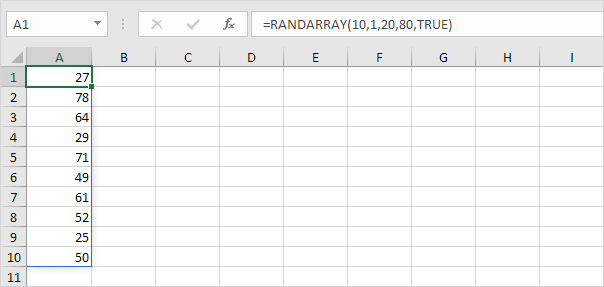Note: the Boolean TRUE (fifth argument) tells the RANDARRAY function to return an array of integers. Use FALSE to return an array of decimal numbers between 20 and 80.

Go to Next Chapter: Round